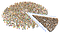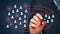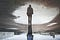# Step by Step to Understanding K-means Clustering and Implementation with sklearn

Simple explanation regarding K-means Clustering in Unsupervised Learning and simple practice with sklearn in pythonsource : How to Use Customer Segmentation in Google Analytics to Build Your Buyer Personal

Previous article :

# Remember Clustering Intuition ?

In the previous article, I was explained regarding Clustering Intuition.

Clustering : grouping data based on similarity patterns based on distance

# Understanding Clustering in Unsupervised Learning

Simple explanation regarding Clustering in Unsupervised Learningsource : Lookalike modeling breathing new life into old channels

# Remember Unsupervised Learning ?

In the previous article, I was explained regarding Unsupervised Learning. Unsupervised Learning is a discovery pattern Given data input only without any label.

According to Wikipedia :

Unsupervised learning is a type of machine learning that looks for previously undetected patterns in a data set with no pre-existing labels and with a minimum of human supervision. In contrast to supervised learning that usually makes use of human-labeled data, unsupervised learning, also known as self-organization allows for modeling of probability densities over inputs. …

# Machine Learning Explanation : Supervised Learning & Unsupervised Learning

What Is Machine Learning? What Is Supervised Learning? and What Is Unsupervised Learning? — Simple explanation regarding Machine Learning

# Part 1 : Regression and Classification Model Evaluation

An introduction and intuition, how evaluate regression and Classification model in generalsource : https://unsplash.com/

# 1. Introduction

Data scientists often use machine learning models to generate insight but wow does a Data scientist make a decision, whether the model will be implemented or not? When the model is implemented, there will be negative and positive impacts on the business. In order to prevent or minimize negative impacts, it is necessary to evaluate the model, so that it can estimate the positive impact and negative impact generated. Is this, model evaluation is one of the most important parts of machine learning.

In this moment, we’ll learn…

# A Simple Introduction to The Random Forest Method

Intuition, Motivation, and How it works on the Random Forest Method

# 1. Introduction

Random Forest lives up to its name : simply, made up of several trees. In more details, Random Forest is a set of decision trees built on random samples with different policies for splitting a node . The implementation, random forest uses the bootstrap method in building decision trees and there are two ways to interpret these results; the more common approach is based on a majority vote in classification case and an average in regression case.

## Related Fundamental knowledge

The ideas behind Random Forest. in fact, are containing so many topics…

# Resampling Methods — A Simple Introduction to The Bootstrap Method

Intuition, motivation, and how it works on the bootstrap method

# Introduction

In statistics, the bootstrap is a widely applicable and extremely powerful statistical tool that can be used to quantify the uncertainty associated with a given estimator or statistical learning method. The bootstrap can derive a strong estimate of a population parameter such as standard deviation, mean, median, standard error, etc.

In wikipedia — the bootstrap is statistical method for estimating the sampling distribution of an estimator by sampling with replacement from the original sample, most often with the purpose of deriving robust estimates of standard errors and confidence intervals of…

# Regression in Decision Tree — A Step by Step CART (Classification And Regression Tree)

Decision Tree Algorithms — Part 3

# 1. Introduction

In previous learning has been explained about The Basics of Decision Trees and A Step by Step Classification in CART, This section will explain A Step by Step Regression in CART.

As has been explained, Decision Trees is the non-parametric supervised learning approach. In addition to classification with continuous data on the target, we also often find cases with discrete data on the target called regression. In the regression, the simple way can be to use Linear Regression to solve this case. …

# Classification in Decision Tree — A Step by Step CART (Classification And Regression Tree)

Decision Tree Algorithms — Part 2

# 1. Introduction

CART (Classification And Regression Tree) is a decision tree algorithm variation, in the previous article — The Basics of Decision Trees. Decision Trees is the non-parametric supervised learning approach. CART can be applied to both regression and classification problems.

As we know, data scientists often use decision trees to solve regression and classification problems and most of them use scikit-learn in decision tree implementation. Based on documentation, scikit-learn uses an optimised version of the CART algorithm

# 2. How Does CART work in Classification

in the previous article it was explained that CART uses Gini Impurity in the process of splitting the…

# The Basics of Decision Trees

Decision Tree Algorithms - Part 1

## 1. Introduction

Decision Trees is the non-parametric supervised learning approach, and can be applied to both regression and classification problems. In keeping with the tree analogy, decision trees implement a sequential decision process. Starting from the root node, a feature is evaluated and one of the two nodes (branches) is selected, Each node in the tree is basically a decision rule. This procedure is repeated until a final leaf is reached, which normally represents the target. Decision trees are also attractive models if we care about interpretability.

## 2. Various Decision Tree Algorithms

There are algorithms for creating decision trees :

# Linear Regression

## Basic of Simple and Multiple Linear Regression

1. Introduction

Linear regression is the supervised learning approach. In particular, linear regression is a useful method for predicting continuous values (target) and attempts to model the linear relationship between target and one or more predictor.

2.Simple Linear Regression (one predictor)

Simple linear regression lives up to its name: simply, finding a relationship between one predictor (𝑥) and target (y). Mathematically, we can write this linear relationship as

In Equation 1, ŷ is target, 𝑥 is Predictor, 𝜷₀ and 𝜷₁ (coefficients) are two unknown constants that represent the intercept and slope terms in the linear model. Simple linear regression attempts to…## Arif R

Data Scientist and Artificial Intelligence Enthusiast